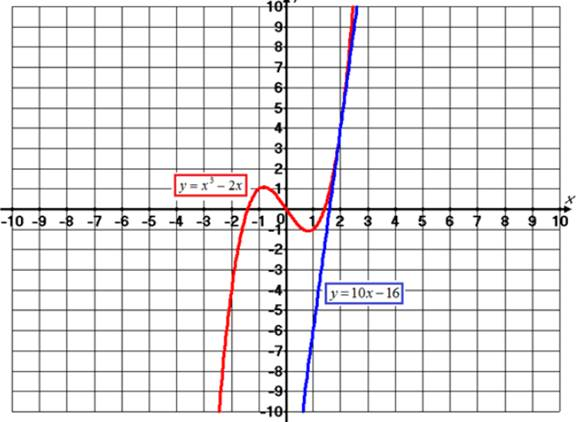# Thevalue of f ' ( 2 ) .### Single Variable Calculus: Concepts...

4th Edition
James Stewart
Publisher: Cengage Learning
ISBN: 9781337687805### Single Variable Calculus: Concepts...

4th Edition
James Stewart
Publisher: Cengage Learning
ISBN: 9781337687805

#### Solutions

Chapter 2, Problem 30RE

(a)

To determine

## To find:Thevalue of f'(2) .

Expert Solution

The value of f'(2) is 10 .

### Explanation of Solution

Given information:

The given function is f(x)=x32x .

Calculation:

Using the derivative.

f'(2)=limh0f(2+h)f(2)h=limh0(2+h)32(2+h)[2'2(2)]h=limh0233(2)3h+3(2)h242h+8+4h=limh0812h+6h242h+8+4h

=limh010h+6h2h=10+6(0)=10

Therefore, the value of f'(2) is 10 .

(b)

To determine

### To find:The equation of tangent line to the curve y=x3−2x at point (2,4) .

Expert Solution

The equation of tangent line to the curve y=x32x at point (2,4) is y=10x16 .

### Explanation of Solution

Given information:

The given function is f(x)=x32x .

Calculation:

The equation of tangent line to the curve y=x32x at point (2,4) is.

yy1=f'(2)(xx1)y4=10(x2)y4=10x20y=10x16

=limh010h+6h2h=10+6(0)=10

Therefore, the equation of tangent line to the curve y=x32x at point (2,4) is y=10x16 .

(c)

To determine

Expert Solution

### Explanation of Solution

Given information:

The given curveare y=x32x and y=10x16 .

Graph:

The graph for the given curve is shown in figure (1).Figure (1)

Interpretation: Graph for the curve y=x32x and y=10x16 is shown in figure (1).

### Have a homework question?

Subscribe to bartleby learn! Ask subject matter experts 30 homework questions each month. Plus, you’ll have access to millions of step-by-step textbook answers!